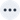# Omitting outer aggregation from your formula

This article discusses the effect of omitting the outer aggregation when using group aggregation. To test for yourself, use the data set we provide in prerequisites.

## Prerequisites

Before attempting the following practice examples, follow these steps to upload the sample dataset:

Click the dropdown to see the steps to upload the dataset.

3. Click the moremenu icon in the upper-right of the screen. From the menu, select Upload data.

4. Upload the data file, either by dragging and dropping it into the field provided, or by clicking Browse your files and selecting Meetings_tiny.csv.

2. To answer Are the fields separated by?, select Comma (,).

5. Select Next at the lower right of the screen to proceed.

6. Select Next to proceed without changing the column names. For the sake of the formulas used in the examples, column names should not be changed.

7. Review the column types in Step 3 and select Upload to finish.

8. Click the search icon on the final screen of the upload process to begin a search based on the sample data set.

## Removing outer aggregation

In this example, we begin with the following formula:

``````average(
group_aggregate(
sum(hours),
query_groups() + {activity classification},
{}
)
)``````

This formula specifies that we should aggregate hours by summing to the level of detail specified by the dimensions in the search bar + activity classification. Then we should re-aggregate to the search bar level of detail by averaging.

Using our Meetings dataset as an example, this would mean that for each account we do the following:

1. Calculate two values:

1. sum of hours for all meetings and

2. sum of hours for all calls.

2. Average those two values to calculate the final value for the account.

The resulting table contains one row per account, containing the averaged value.

To test this with our sample dataset, follow these steps:

2. Enter `hours` `activity classification` in the search bar and select Go.

3. Click the more options iconand select Add formula.

4. In the formula pop-up window, name your formula. Here, we have named the formula average hours per activity classification.

5. Enter the following formula and select Save:

``````average(
group_aggregate(
sum(hours),
query_groups() + {activity classification},
{}
)
)``````
6. Search for: `account` `average hours per activity classification` `sort by account`.

7. The following table results:

Table 1. Example 5.1.1
Account Hours per activity classification averaged

Amazon

11

Oracle

18

Redshift

3

Snowflake

3.5

In any formula, you have the option of omitting the outer aggregation ("average" in this case). We do not recommend omitting outer aggregation, as it may lead to confusing results. When you omit the outer aggregation, the result shows the table or chart at the level of detail of the inner aggregation. In this example, the inner aggregation is at the activity classification level of detail.

If we omit the outer aggregation, the table displays as follows:

1. Search for: `account` `average hours per activity classification` `sort by account`.

2. The following table results:

Table 2. Example 5.1.2
Account Hours per activity classification averaged

Amazon

11

Oracle

18

Redshift

5

Redshift

1

Snowflake

2

Snowflake

5

In the preceding table, because the outer aggregation has been omitted from the hours per activity classification averaged formula, we display the table at the activity classification level of detail, but we do not show a column for activity classification.

3. If you add an activity classification column, this table makes more sense:

1. Search for: `account` `average hours per activity classification` `sort by account`.

2. The following table results:

Table 3. Example 5.1.3
Account Activity classification Hours per activity classification averaged

Amazon

meeting

11

Oracle

meeting

18

Redshift

meeting

5

Redshift

call

1

Snowflake

meeting

2

Snowflake

call

5

In a nutshell, omitting the outer aggregation means the formula does not aggregate to the level of detail specified in the search bar. To avoid confusion, we recommend including outer aggregation.

If you prefer to omit the outer aggregation from the formula, you can place your outer aggregation in the search bar. For example, if you omit the outer aggregation in the previous example’s formula, the resulting formula would look like this:

Hours per activity classification averaged
``````group_aggregate(
sum(hours),
query_groups() + {activity classification},
{}
)``````

In this case, you could achieve outer aggregation to the search level of detail by putting the following in your search:

1. Search for: `account` `average hours per activity classification` `sort by account`

Table 4. Example 5.1.4
Account Average hours per activity classification

Amazon

11

Oracle

18

Redshift

3

Snowflake

3.5

This results in the same table generated by including the outer aggregation in the formula, but the column heading name begins with "Average".

This is a valid approach, but requires that the user of the formula remember to add the aggregation to the front of the formula when used in the search bar. When omitted, the results are confusing, so we recommend you always include the outer aggregation in the formula.

Related information: